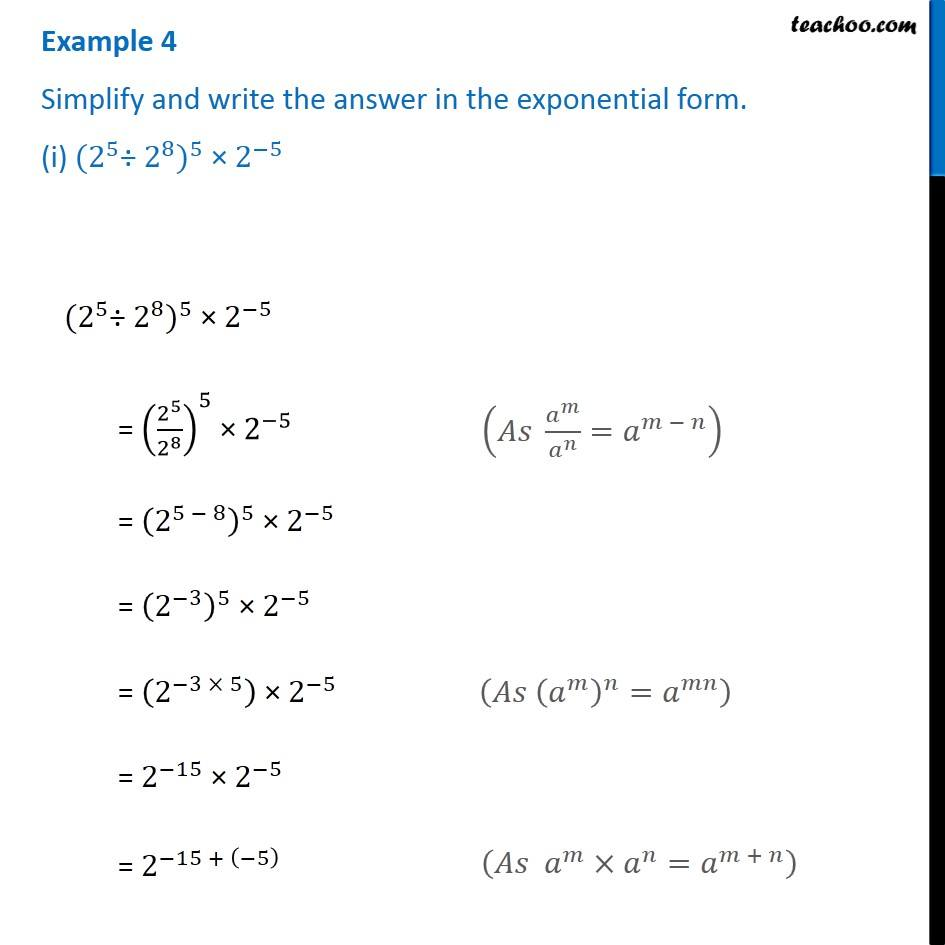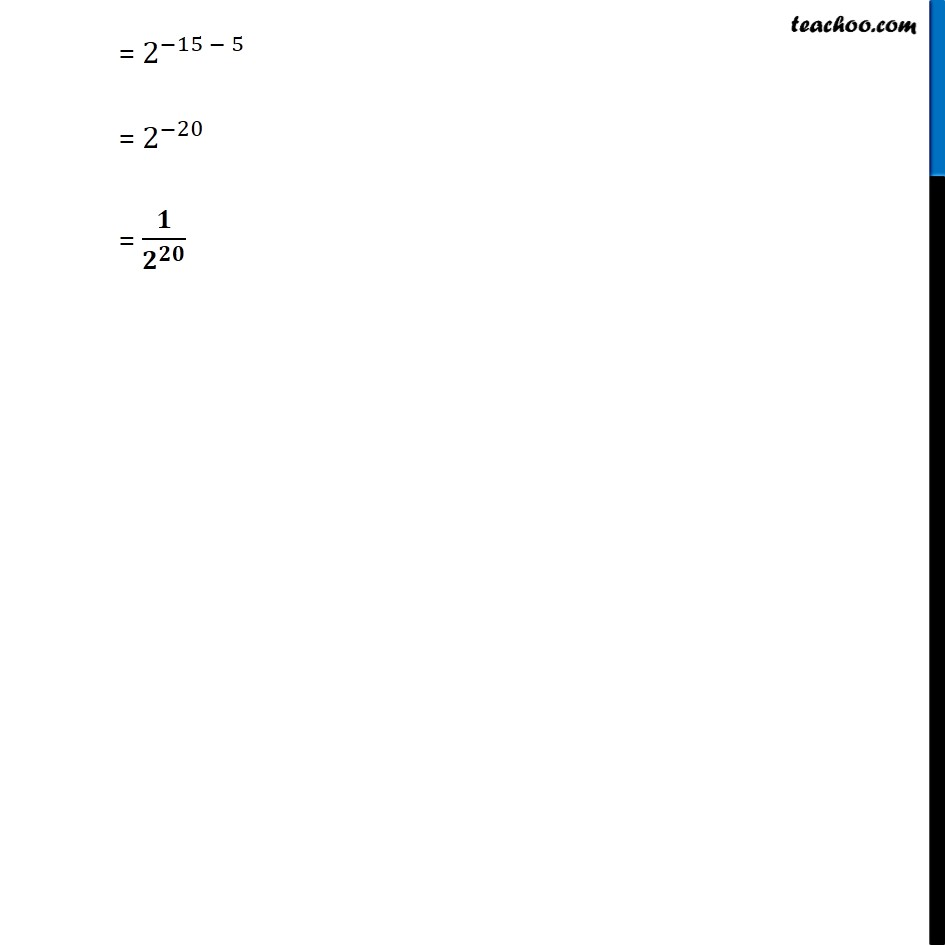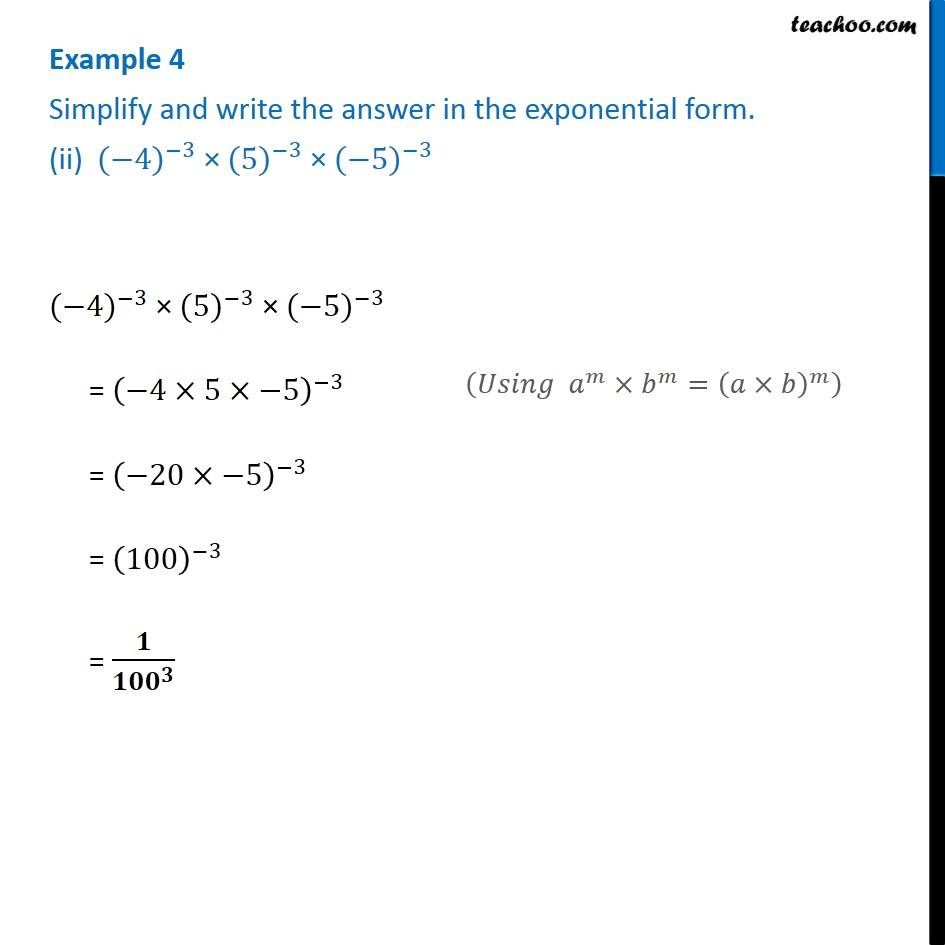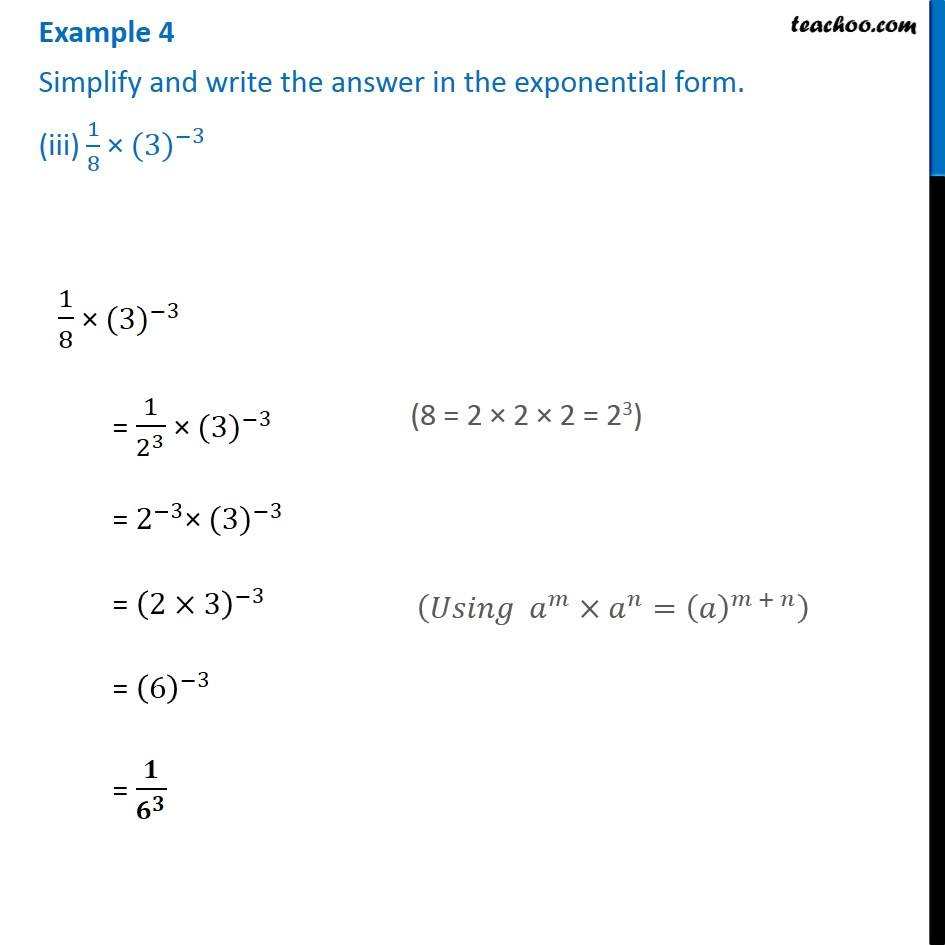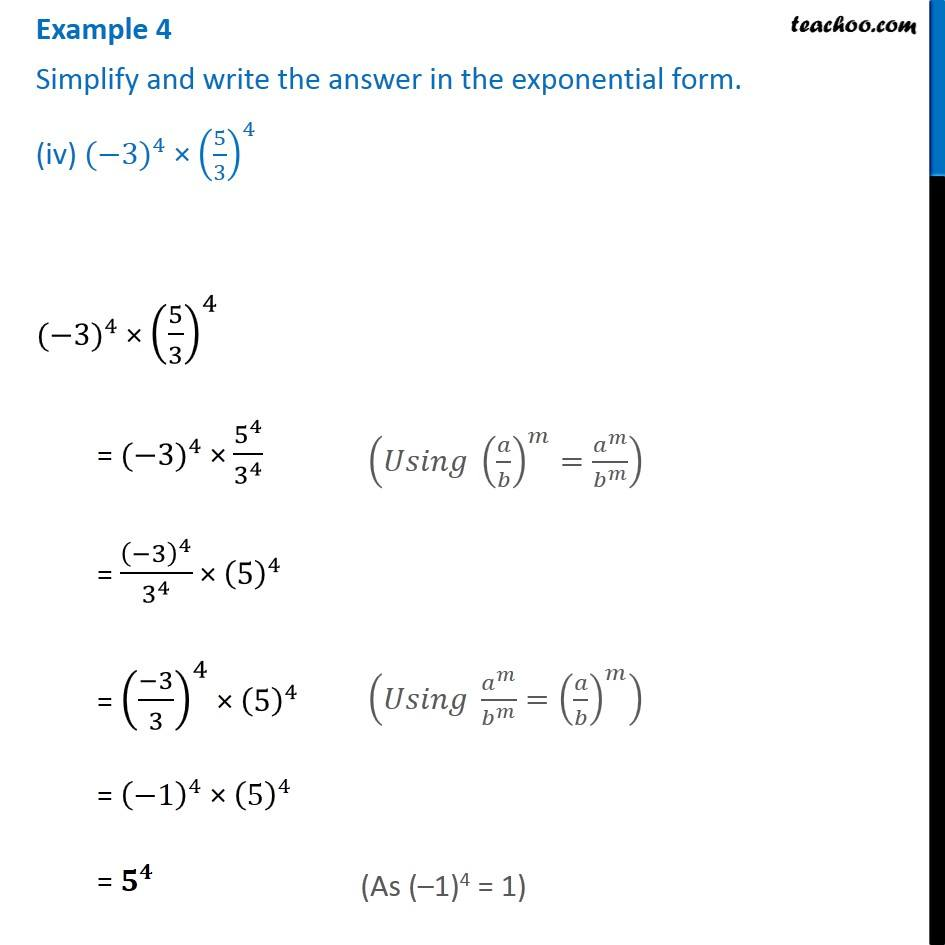Subscribe to our Youtube Channel - https://you.tube/teachoo

1. Chapter 12 Class 8 Exponents and Powers
2. Serial order wise
3. Examples

Transcript

Example 4 Simplify and write the answer in the exponential form. (i)〖〖 (2〗^5 "÷ " 2^8)〗^5 × 2^(−5) 〖〖 (2〗^5 "÷ " 2^8)〗^5 × 2^(−5) = (2^5/2^8 )^5 × 2^(−5) = (2^(5 − 8) )^5 × 2^(−5) = (2^(−3) )^5 × 2^(−5) = (2^(−3 × 5) ) × 2^(−5) = 2^(−15) × 2^(−5) = 2^(−15 + (−5) ) (𝐴𝑠 𝑎^𝑚/𝑎^𝑛 =𝑎^(𝑚 − 𝑛) ) (𝐴𝑠 (𝑎^𝑚 )^𝑛=𝑎^𝑚𝑛 ) (𝐴𝑠 𝑎^𝑚×𝑎^𝑛=𝑎^(𝑚 + 𝑛) ) = 2^(−15 − 5) = 2^(−20) = 𝟏/𝟐^𝟐𝟎 Example 4 Simplify and write the answer in the exponential form. (ii) 〖(−4)〗^(−3) × 〖(5)〗^(−3) × 〖(−5)〗^(−3) 〖(−4)〗^(−3) × 〖(5)〗^(−3) × 〖(−5)〗^(−3) = (−4×5×−5)^(−3) = (−20×−5)^(−3) = (100)^(−3) = 𝟏/〖𝟏𝟎𝟎〗^𝟑 (𝑈𝑠𝑖𝑛𝑔 𝑎^𝑚×𝑏^𝑚=(𝑎×𝑏)^𝑚 ) Example 4 Simplify and write the answer in the exponential form. (iii) 1/8 × 〖(3)〗^(−3) 1/8 × 〖(3)〗^(−3) = 1/2^3 × 〖(3)〗^(−3) = 2^(−3)× 〖(3)〗^(−3) = (2×3)^(−3) = (6)^(−3) = 𝟏/𝟔^𝟑 (8 = 2 × 2 × 2 = 23) (𝑈𝑠𝑖𝑛𝑔 𝑎^𝑚×𝑎^𝑛=(𝑎)^(𝑚 + 𝑛) ) Example 4 Simplify and write the answer in the exponential form. (iv) 〖(−3)〗^4 × (5/3)^4 〖(−3)〗^4 × (5/3)^4 = 〖(−3)〗^4 × 5^4/3^4 = (−3)^4/3^4 × (5)^4 = ((−3)/3)^4 × (5)^4 = (−1)^4 × (5)^4 = 𝟓^𝟒 (𝑈𝑠𝑖𝑛𝑔 (𝑎/𝑏)^𝑚=𝑎^𝑚/𝑏^𝑚 ) (𝑈𝑠𝑖𝑛𝑔 𝑎^𝑚/𝑏^𝑚 =(𝑎/𝑏)^𝑚 ) (As (–1)4 = 1)

Examples